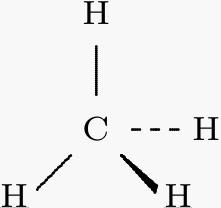# What is the orbital hybridization theory?

Jul 2, 2014

Orbital hybridization is the concept of mixing atomic orbitals to form new hybrid orbitals. These new orbitals have different energies, shapes, etc., than the original atomic orbitals. The new orbitals can then overlap to form chemical bonds.

An example is the hybridization of the carbon atom in methane, CH₄. We know that all four C-H bonds in methane are equivalent. They point towards the corners of a regular tetrahedron with bond angles of 109.5°.Thus, the carbon must have four orbitals with the correct symmetry to bond to the four hydrogen atoms.

The ground state configuration of a carbon atom is $1 {s}^{2} 2 {s}^{2} 2 {p}_{x} 2 {p}_{y}$.We can see this configuration on the left hand side of the diagram above.

The carbon atom can use its two singly occupied p orbitals to form two covalent bonds with two hydrogen atoms, yielding ·CH₂·. This is not methane.

The carbon atom can also excite an electron from the 2s orbital to the empty 2p orbital, as in the dotted line above. This gives four singly occupied orbitals.

The C-H bonds formed by overlap with the three 2p orbitals would have bond angles of 90°. The C-H bond formed by overlap with the 2s orbital would be at some other angle. This is not the structure of methane.

If the 2s and the 2p orbitals are mathematically mixed ("hybridized"), we get four new equivalent sp³ orbitals. The math predicts the bond angles to be 109.5° — just what we see in methane.The energy released by formation of four bonds over-compensates for the excitation energy required. Thus, the formation of four C-H bonds is energetically favoured.

Hope this helps.